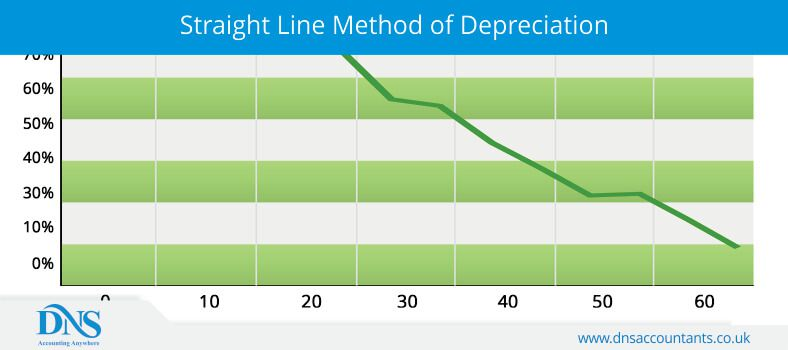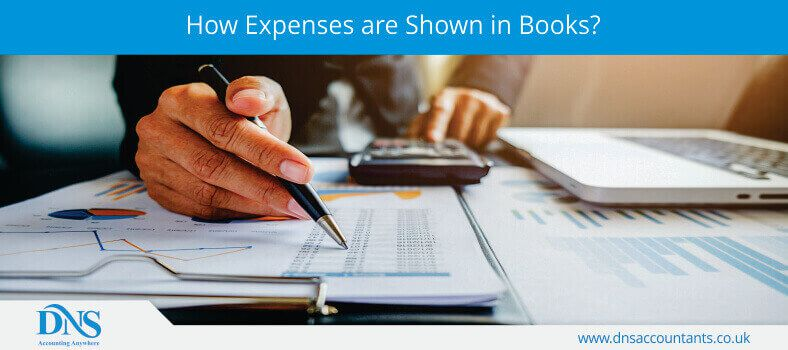# Calculate Straight Line Depreciation using Simple FormulaTo define depreciation of any machine would be to say that the wear and tear caused to the machine and its part in the process of natural and regular use is called as depreciation. Usually, depreciation of any kind points towards only one thing,and that is the decrease in the value of the machine over time. Every machine is said to depreciate every day, and that depreciation can be calculated with the help of some specific formulas. However, the value of depreciation and the percentage of the value that depreciates for a particular machine vary largely on the type of the machine it is. Some machines even witness 100% depreciation in a matter of a year.

#### What is a straight-line method of depreciation?The straight line method of depreciation is one of the most widely used and popular methods of calculation of depreciation expenses. The popularity of this method comes because of the ease of use it provides and the uniform nature of the depreciation method.

In this method, the cost of a fixed asset is uniformly decreased over the span of its regular life. This depreciation happens at a uniform rate and at a uniform price year after year. Since the charged expense as depreciation is the same every year, the value of the fixed asset declines in uniform line over the course of years.

The formula for calculation of depreciation for straight-line basis is given under:

Straight-line Method = Depreciable Amount/Useful Life

The above-mentioned formula can be used to calculate the amount of the expense that has to be charged as depreciation for any kind of formula. To come to the legitimate price or amount that is to be charged, a person will require to only input the values in the formula. For Depreciable Amount, the amount or the price of the machinery will be added and for Useful Life will be the expected time of use of the machine or for how long will the machine work.

#### What is depreciation?

In simple terms, depreciation is the decrease in the value of the machine due to the normal wear and tear arising out of use or out of non-use of the machine. This value, the decrease in the actual value of the machine or machinery can be calculated in monetary terms and expressed in same.

Depreciation is mainly a term used in accountancy. This term allows businesses firstly, to relocate the cost of the machine to other areas of the business according to the actual lifespan of the machine. And secondly, depreciation is a way to decrease the tax burden of the business. The expense or the depreciation charges are shown on the balance sheet as “depreciation expenses” or just “depreciation.” This expense reduces the amount of net profit and decreases the tax liability of the business. Depreciation should be calculated by small business owners who own assets related to his business, landlords dealing with properties, buy-to-let, contractors owning machines and construction industry firm's owner.

#### What are the types of Depreciation?

There are various methods of calculating and computing depreciation of any given machinery or machinery. These methods can be opted by the business or the company as per their discretion and the type of machine they intend to use. Here is a list of four broad types of depreciation evaluation methods:

• Straight-line
• Double declining method
• Sum of years and
• Units of production

All of these methods make use of different kinds of formulas and take different factors into consideration to come with the perfect amount of the depreciation expense that is to be charged.

#### How are depreciation costs and expenses governed?

Depreciation is a natural occurrence. However, the fact that various kinds of methods for calculation and various kinds of methods for such calculation exist in this world, it becomes hard to understand which method is to be used and where it should be used. However, to keep a clear understanding, there are many accounting standards and many rules as per which such charges and expenses are to be calculated and charged against profit. Every country has a different method of charging such expenses.

In the UK, all of the UK accounting standards from August 1990 was issued by the Accounting Standards Board (ASB). However, in mid of 2012, the rules and board specifying the standards changed and the power for creation and regulation of such standards was given to FRC Board. All standards developed and specified in the UK for accounting purposes is known as Financial Reporting Standards (FRSs).

#### Will I get any benefits of showing depreciation expenses for my profit statements?

One of the biggest concerns for depreciation and its charges is the question of any benefit to avail out of showing or charging depreciation expenses to profits.

Well, as mentioned before, there are two major benefits of expressing the expenses for depreciation against profits. However, there is yet another benefit of doing so. Depreciation has been used by businesses for a long a time as one of the legitimate ways and methods to retain the actual profits of the business. With depreciation expenses charged every year, the cost of such depreciation is disturbed among the owners of the business.

This practice allows the business to retain profits in the business and it creates a fund that can be used by the owners at any point to purchase new machinery without spending too much money. Other than this, the reserve can be used for other purposes, as deemed fit by the owners and by the law.

#### How are these expenses shown in the books?At present, the use of software for accounting has made accounting simple, and the entries for various expenses have become automated. However, earlier, when software for such entries did not exist, these entries were first entered in the journals of the business and then transferred to the balance sheet and would be deducted from the actual or the remaining value of the machine.

Here is how the journal entries for depreciation look when entered manually:

Depreciation Expense xxx
Accumulated Depreciation xxx

The entries for the depreciation of journal entries for each year would be the same, including the amount that has to be charged. So, this entry can be copied directly to the books at the end of each year until the last year of the machine’s useful life.

#### How to use accounting software to find out the depreciation expense?

Setting up the software for calculating the depreciation expense has some points to be noted and remembered before you can find the actual amount of depreciation:

• While setting up the profile for depreciation of the machine of your choice, remember to select the single-line method of calculation. This will allow your software calculate the amount for depreciation and keep it uniform for the years to come.
• Next, you need to select a depreciation year. A person can either select a fiscal year or a calendar year for such calculation. Depreciation for a year will always be charged from the date of purchase. For example, if you purchase machinery in June and your business makes use of the calendar year, then your calculation will only be from the month of June to December. The calculation of a number of days, months is to be taken strictly while computing depreciation to attain a fair and true value.
• Utmost care has to be taken when you post your entries. The monthly and yearly postings are to spelled out clearly.

#### What can all be considered for depreciation?

There are many things and objects that can be depreciated, and here is a list of such objects and things:

• new HVAC system
• roof replacement
• landscaping improvements
• capital improvements
• leasehold improvements
• equipment used to maintain the property
• new windows

However, one cannot depreciate the costs of service contracts and other time-bound contracts; you can only depreciate tangible assets.

#### How Long Does Depreciation Last?

The cost of depreciation cannot go on forever. However, there are some rules to be followed by the accountants. Buildings can be depreciated for over 39 years. However, other standard machines and tangible assets can be depreciated for about 5 to 7 years.

#### What Happens If I Sell The Property?

Another kind of question that arises in our minds while talking about depreciation is that what would happen if a property is being sold? A building is depreciated even though the land is not. However, the depreciation expenses will be considered and used to find out the adjusted cost of the building,and the cost at which the building was sold will be considered as the selling price of the building.

Now, in such as the case you will have to pay tax on a dual basis. One for the adjusted cost of the building and one against the profit made by the seller or the owner of the building as per the cost of building at its initial purchase by the owner deducted by the sum of money at which the building was sold at.

#### Tag

straight line depreciation formula excel  depreciation straight line method questions and answers  straight line depreciation definition  double declining depreciation formula  depreciation straight line method questions and answers pdf  depreciation formula math  depreciation formula accounting  reducing balance depreciation

Speak with an expert

Any questions? Schedule a call with one of our experts.